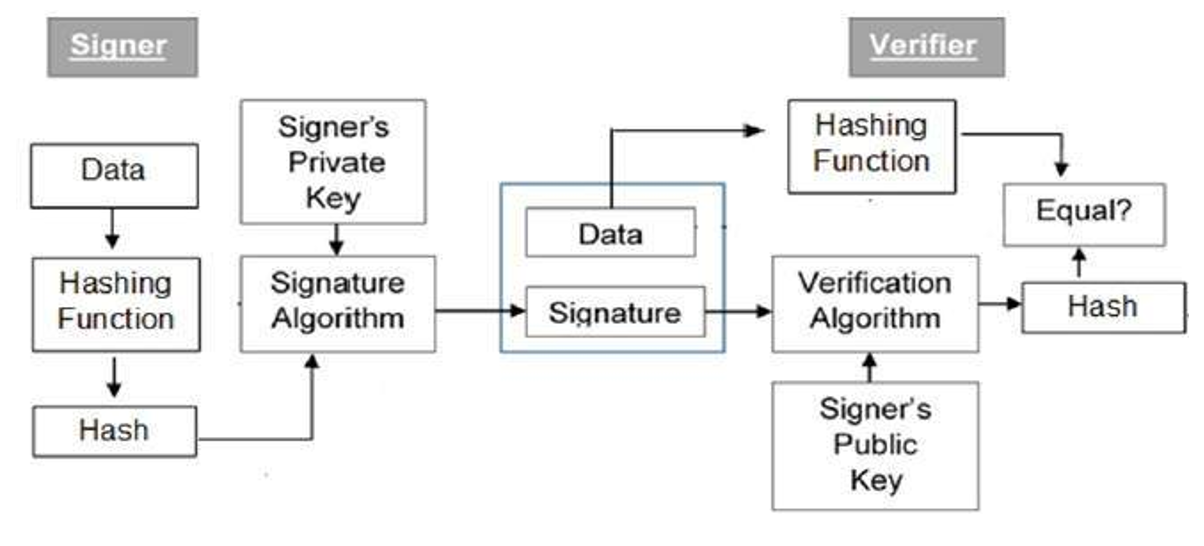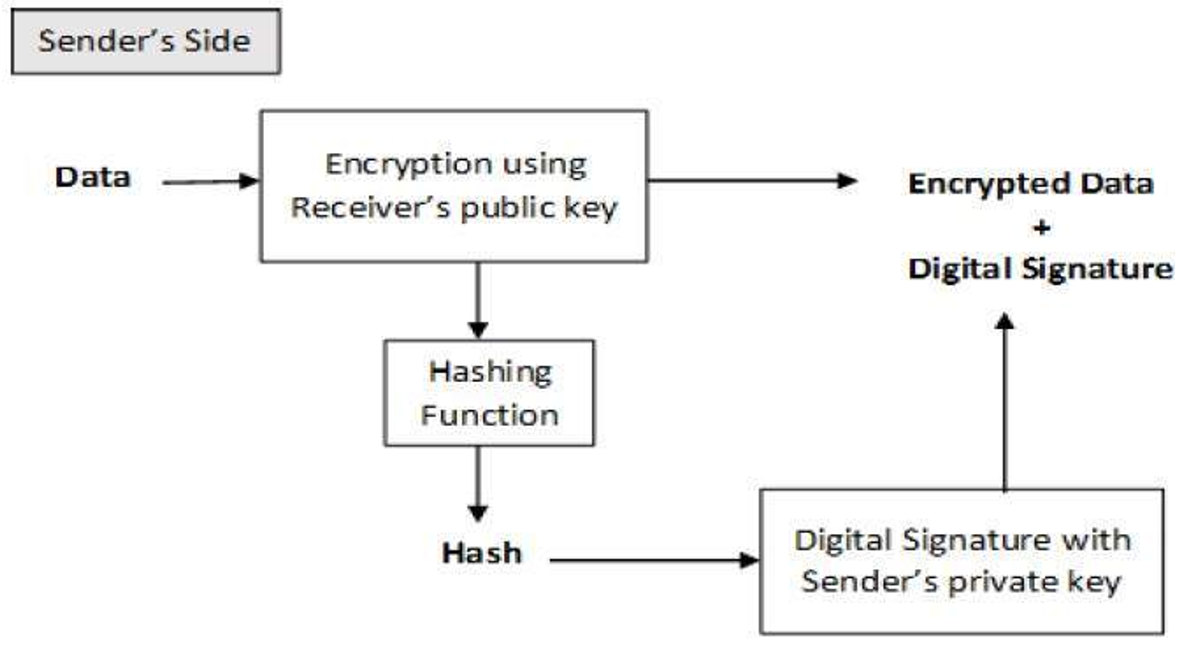# Digital Signature Algorithm (DSA) in Cryptography

Cryptography | Digital Signature Algorithm (DSA): In this tutorial, we will learn about the digital signature algorithm (DSA), its steps of encryption and decryption, and the types of messages in the Digital Signature Algorithm. By Monika Sharma Last updated : May 25, 2023

## Digital Signature Algorithm (DSA)

Digital Signature Algorithm (DSA) is one of the Federal Information Processing Standard for making digital signatures depends on the mathematical concept or we can say the formulas of modular exponentiation and the discrete logarithm problem to cryptograph the signature digitally in this algorithm.

It is Digital signatures are the public-key primitives of message authentication in cryptography. In fact, in the physical world, it is common to use handwritten signatures on handwritten or typed messages at this time. Mainly, they are used to bind signatory to the message to secure the message.

Therefore, a digital signature is a technique that binds a person or entity to the digital data of the signature. Now, this will binding can be independently verified by the receiver as well as any third party to access that data.

Here, Digital signature is a cryptographic value that is calculated from the data and a secret key known only by the signer or the person whose signature is that.

In fact, in the real world, the receiver of message needs assurance that the message belongs to the sender and he should not be able to hack the origination of that message for misuse or anything. Their requirement is very crucial in business applications or any other things since the likelihood of a dispute over exchanged data is very high to secure that data.

## Block Diagram of Digital Signature

The digital signature scheme depends on public-key cryptography in this algorithm.### Explanation of the block diagram

• Firstly, each person adopting this scheme has a public-private key pair in cryptography.
• The key pairs used for encryption or decryption and signing or verifying are different for every signature. Here, the private key used for signing is referred to as the signature key and the public key as the verification key in this algorithm.
• Then, people take the signer feeds data to the hash function and generates a hash of data of that message.
• Now, the Hash value and signature key are then fed to the signature algorithm which produces the digital signature on a given hash of that message. This signature is appended to the data and then both are sent to the verifier to secure that message.
• Then, the verifier feeds the digital signature and the verification key into the verification algorithm in this DSA. Thus, the verification algorithm gives some value as output as a ciphertext.
• Thus, the verifier also runs the same hash function on received data to generate hash value in this algorithm.
• Now, for verification, the signature, this hash value, and output of verification algorithm are compared with each variable. Based on the comparison result, the verifier decides whether the digital signature is valid for this or invalid.
• Therefore, the digital signature is generated by the 'private' key of the signer and no one else can have this key to secure the data, the signer cannot repudiate signing the data in the future to secure that data by the cryptography.

## Importance of Digital Signature

Therefore, all cryptographic analysis of the digital signature using public-key cryptography is considered a very important or main and useful tool to achieve information security in cryptography in cryptoanalysis.

Thus, apart from the ability to provide non-repudiation of the message, the digital signature also provides message authentication and data integrity in cryptography.

This is achieved by the digital signature are,

• Message authentication: Therefore, when the verifier validates the digital signature using the public key of a sender, he is assured that signature has been created only by a sender who possesses the corresponding secret private key and no one else does by this algorithm.
• Data Integrity: In fact, in this case, an attacker has access to the data and modifies it, the digital signature verification at the receiver end fails in this algorithm, Thus, the hash of modified data and the output provided by the verification algorithm will not match the signature by this algorithm. Now, the receiver can safely deny the message assuming that data integrity has been breached for this algorithm.
• Non-repudiation: Hence, it is just a number that only the signer knows the signature key, he can only create a unique signature on a given data of that message to change in cryptography. Thus, the receiver can present data and the digital signature to a third party as evidence if any dispute arises in the future to secure the data.

## Encryption with Digital Signature

It is desirable to exchange encrypted messages than plaintext to achieve confidentiality in cryptography. In fact, in the public key encryption scheme, this is a public or encryption key of the sender is available in the open domain, and hence anyone can spoof his identity and send an encrypted message to the receiver in this algorithm.## DSA Algorithm Steps

The first part of the DSA algorithm is the public key and private key generation through some steps, which can be told as:

• Firstly, choose a prime number q, which is called the prime divisor in this.
• Then, choose another primer number p, such that p-1 mod q = 0. p is called the prime modulus in this.
• Then, choose an integer g, such that 1 < g < p, g**q mod p = 1 and g = h**((p–1)/q) mod p. q is also called g's multiplicative order modulo p in this algorithm.
• Then, choose an integer, such that 0 < x < q for this.
• Now, compute y as g**x mod p.
• Thus, Package the public key as {p,q,g,y} is this.
• And, Package the private key as {p,q,g,x} is this.

Then, the second part of the DSA algorithm is the signature generation and signature verification in this algorithm, which can be told as:

Firstly, to generate a message signature, the sender can follow these further steps:

• Firstly, generate the message digest h, using a hash algorithm like SHA1.
• Then, generate a random number k, such that 0 < k < q.
• Then, Computer as (g**k mod p) mod q. If r = 0, select a different k.
• And, Compute i, such that k*i mod q = 1. i is called the modular multiplicative inverse of k modulo q in this.
• Then, Compute s = i*(h+r*x) mod q. If s = 0, select a different k.
• Thus, Package the digital signature as {r,s}.

Then, to verify a message signature, the receiver of the message and the digital signature can follow these further steps as:

• Firstly, Generate the message digest h, using the same hash algorithm.
• Then, Compute w, such that s*w mod q = 1. w is called the modular multiplicative inverse of s modulo q in this.
• Then, Compute u1 = h*w mod q.
• And, Compute u2 = r*w mod q.
• Then, Compute v = (((g**u1)*(y**u2)) mod p) mod q.
• Wherever, If v == r, the digital signature is valid.

Image Reference: https://www.tutorialspoint.com/cryptography/cryptography_digital_signatures.htm